# Discriminant Calculator

A discriminant calculator can be used for finding the quadratic equation's discriminant value for the specified coefficients.

$$a x^2 + bx + c = 0$$

## Discriminant Formula for Quadratic Equation

The discriminant formula for the quadratic equation $$ax^2 + bx + c = 0$$, where $$a ≠ 0$$, is $$b^2 – 4ac$$.

The discriminant is also represented by the symbol $$Δ$$.

$$∴ Δ = b^2 – 4ac$$

Calculations are made quicker and easier using the online discriminant calculator tool from Mathematics Master, which shows the result in a split second.

 Graphs of $$y = ax^2 + bx + c$$ Discriminant Number of types and solutions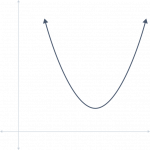Discriminant is negative No x-intercepts No Roots Two imaginary solutions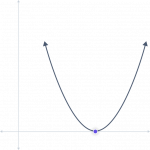Discriminant is zero One x-intercept One Root (a double root) One real solution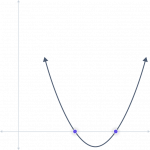Discriminant is positive Two x-intercepts Two Roots Two real solutions

## What is the Discriminant?

The discriminant describes the characteristics of quadratic equations’ roots. The equations can distinguish between possible types of answers, including:

• In the case of a positive discriminant value, we obtain two real solutions.
• There is just one real solution when the discriminant value is zero.
• In the case of negative discriminant value, we have two complex solutions.

## What is Discriminant in Math?

The value that assists us in identifying the different types of solutions when using the quadratic formula is known as the “discriminant.” It is a unique function of a quadratic equation’s coefficient whose value provides details about its roots.

The discriminant distinguishes three distinct sorts of quadratic equation solutions.

Case 1: If the discriminant D exceeds 0, we can take the square root and get two valid answers.

Consider a=2, b=5, c=3

$$=b^2-4ac$$

$$=(5)^2-4(2)(3)$$

$$=25-24$$

$$= 1$$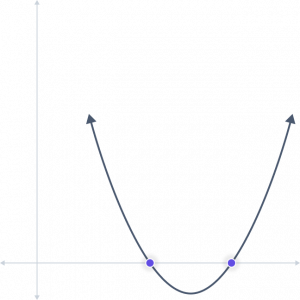Case 2: There is only one real solution if the discriminant D equals 0, which may be found by taking the square root of 0.

Consider a=4, b=4, c=1

$$=b^2-4ac$$

$$=(4)^2-4(4)(1)$$

$$=16-16$$

$$= 0$$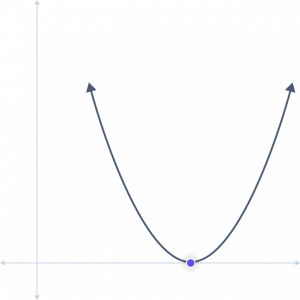Case 3: If the discriminant D is smaller than 0, we can get two complex solutions by taking the square root of a negative number.

Consider a=1, b=4, c=6

$$=b^2-4ac$$

$$=(4)^2-4(1)(6)$$

$$=16-24$$

$$= -8$$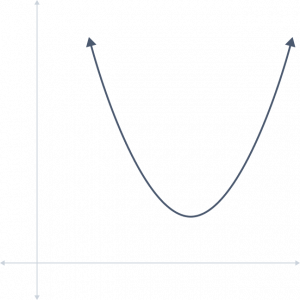## How to Find Discriminant using the Discriminant Calculator?

Take the actions listed below to use the discriminant calculator:

1. Choose the polynomial’s degree first before moving on. You can select polynomials ranging from 2 to 5, including quadratic, cubic, quartic, and quintic polynomials (degrees 2, 3, 4, and 5, respectively).
2. Choose second as the degree, for instance, if your purpose is to find the discriminant of a quadratic equation.
3. Include all of your polynomial coefficients, even the zero-valued ones.
4. To obtain the output, now select “Solve.”
5. The output field will show the discriminant value.

## FAQs

What do the discriminants (Δ) tell us?

With the discriminant, we can determine if there are two solutions, one solution, or no solutions.

How many solutions are possible if the discriminant is more significant than zero?

If the square is perfect, there are two solutions (2 real solutions); otherwise, there are two rational roots and two irrational roots.

Why is the discriminant value in a quadratic equation significant?

The discriminant value reveals the characteristics of the quadratic equation’s roots. The quadratic equation can have real or complex roots. It aids in determining an equation’s solution.